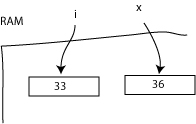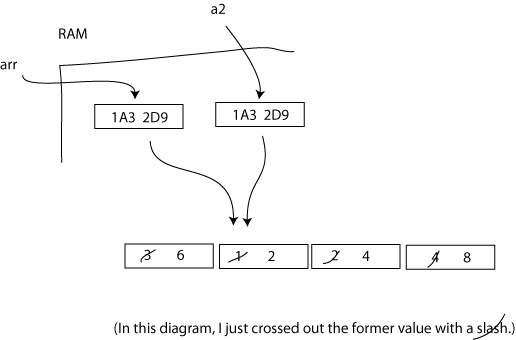Logout

Literal Variables vs Reference Variables: Implications for Parameter Sending

One of the important implications of objects being references is that when they are "sent" as parameters, what is copied by the receiving method is the address, not the whole object. This is a boon for memory efficiency, since the object data does not have to be copied, which would be a waste; only the 32 bits of the address needs to be copied.

Literal Vs Reference Variables

A literal variable is any variable that is literally what we assign it. So in Java, primitive types are literals. If we go int x = 3, then x literally points to 32 bits in memory which are literally the binary equivalent of 3, which is 00000000 00000000 00000000 00000011.

A reference variable is any variable which is an address which points to the data it is there to represent. Objects in Java are therefore reference variables, as are array objects in Java. What they are is references to address. If we go int [] arr = new int , arr is the binary equivalent of an address like 1A2 3D6. And that memory address is the first address of 10 x 32 bits which will store the array of 10 ints.

So since literal variables (in our Java world, Java primitives), and reference variables (in our Java world, structures such as arrays and objects), are thus different, when they are sent as parameters, there are different implications.

Consider the following code segments.

Parameter Sending of (Literal) Primitives

ForParameterSending.java
``` 1
2 package javaapplication67;
3
4 public class ForParameterSending {
5     public static void main(String[] args) {
6         int i = 33;
7         System.out.println("From the main method BEFORE the call to method1, i is: " + i);
8         method1(i);
9         System.out.println("From the main method AFTER the call to method1, i is still: " + i);
10         //The point is that the method does not affect the value of i
11         //Because i is a literal value and is different than x in method1.
12     }
13
14     public static void method1(int x){
15         x+=3;
16         System.out.println("From within the method, x is: " + x);
17     }
18
19 }
20
21
```

Output:

From the main method BEFORE the call to method1, i is: 33.
From the method itself, x is: 36.
From the main method AFTER the call to method1, i is still 33.------------

i remains the same value before and after the call. This is because x and i are two different things in memory, and they are literals; they are the values which they are, not other values that they point to. So it doesn't matter what happens to x in the method; it is different than i, and so i remains unchanged.

Parameter Sending of (Reference) Objects

****Rather than get complicated and make a template class for making this point, I'll just use arrays, which are the same as objects in that their variables are actually reference variables.****

ForObjectParameterSending.java
``` 1
2 package javaapplication67;
3
4
5 public class ForObjectParameterSending {
6     public static void main(String[] args) {
7         int [] arr = {3, 1, 2, 4};
8         System.out.println("The array BEFORE the call to the method.");
9         for(int i = 0; i < arr.length; i++){
10             System.out.print(arr[i] + ", ");
11         }
12         System.out.println("");
13         doubleValuesInTheArray(arr);
14         System.out.println("The call AFTER the call to the method.");
15         for(int i = 0; i < arr.length; i++){
16             System.out.print(arr[i] + ", ");
17         }
18         //The point is that in this case, arr and a2 may be different, but they
19         //are copies of the same address, so they point to the same thing, and
20         //if that thing is changed, it is changed.
21     }
22
23     public static void doubleValuesInTheArray(int [] a2){
24         for(int i = 0; i < a2.length; i++){
25             a2[i]*=2;
26         }
27         System.out.println("The array from within the method:");
28         for(int i = 0; i < a2.length; i++){
29             System.out.print(a2[i] + ", ");
30         }
31         System.out.println("");
32
33     }
34 }
35
36
```

Output:

The array BEFORE the call to the method.
3, 1, 2, 4,
The array from within the method:
6, 2, 4, 8,
The call AFTER the call to the method.
6, 2, 4, 8,-------------------

The content of the first array is changed after the call to the method. Note that what we are printing out here is the data content of the arrays, rather than the array variables themselves. Arrays are objects, and objects are references, so, yes, arr and a2 are indeed different things in memory, but they are copies of the same reference address. So they point to the same thing. What is being changed in the code segment above is the content of the array, rather than the reference itself. Since the reference address of both arr and a2 are the same, if what one is pointing to changes, that is what the other is pointing to also, so what both point to has indeed been changed.

Getting back to the point made at the beginning, having a system where data structures are pointed to with reference variables has a couple of main advantage. One is that you can create structures like linked lists and binary trees. But the other advantage - which has been the focus of this page of notes - is that the variables representing those data structures can be passed around with ease, and not take up un-needed memory. Consider if we had an array with a million elements; if we made a copy of all of those elements when sending it to a method, that would take up and extra million times the size of each element. Whereas since an array is an object, we just send that 32 bit reference.